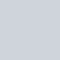# 看完這篇文章你會得到的成果圖# 前言【PyQt5】Day 5 – 開始來設計我們的 controller.py，改以「程式角度」來說明如何建立 PyQt 的系統

# 此篇文章的範例程式碼 github

https://github.com/howarder3/ironman2021_PyQt5_photoshop/tree/main/day16_mouse_get_pos

## 我們先來分析「處理圖片」與「顯示圖片」不一致的問題

• 顯示的圖片 self.qpixmap
• 處理中的圖片 self.img

## 分析兩者之間的「程式」關係

### 1. self.img 是由 OpenCV 的 imread 取得的圖片 (讀入的原圖)

``````self.img = cv2.imread(self.img_path)
``````

### 2. 我們會先經由以下處理，將他轉為 Qimg

``````self.qimg = QImage(self.img, self.origin_width, self.origin_height, bytesPerline, QImage.Format_RGB888).rgbSwapped()
``````

### 3. 再來會由 Qimg 轉為 QPixmap

``````self.origin_qpixmap = QPixmap.fromImage(self.qimg)
``````

### 4. Qpixmap 可能會經由一些縮放的處理，最後藉由 Qlabel 顯示在畫面上

``````self.label_img.setPixmap(self.qpixmap)
``````

### 分析這個流程，發現實際上圖片經過了很多次的轉換，才到最後顯示的部分。

``````OpenCV image -> Qimg -> QPixmap -> Qlabel顯示
``````

• QPixmap 現在的長寬 (會因為顯示而改變)
• QPixmap 與 OpenCV image 的比例差距 (會因為顯示而改變)
• OpenCV image 原圖的長寬 (永遠不變)

``````「QPixmap 現在的長寬」=「OpenCV image 的長寬」*「QPixmap 與 OpenCV image 的比例差距」
``````

## 有沒有更不容易混淆的做法? – 不如我們都「正規化」一下

• 如下圖：我們原來的作法• 如下圖：我們優化的作法 (正規化)# UI 設計部份 (UI.py)

*「點擊座標(顯示圖片的座標)」：label_click_pos
*「換算後，正規化的座標」：label_norm_pos
*「實際座標(對應到原圖片的座標)」：label_real_pos## 轉換成 UI.py

### 轉換 day16.ui -> UI.py

``````pyuic5 -x day16.ui -o UI.py
``````

## 執行看看 UI.py 畫面是否如同我們想像

• 看看我們製作出來的介面
``````python UI.py
``````# controller 設計部份 (controller.py)

## 從 UI.py 中找出物件名稱

*「點擊座標(顯示圖片的座標)」：label_click_pos
*「換算後，正規化的座標」：label_norm_pos
*「實際座標(對應到原圖片的座標)」：label_real_pos

## 同 day13 的 scrollArea 說明，我們一樣需要刪除 scrollAreaWidgetContents 的部份

• 新增與調整的 scrollArea 片段
``````self.scrollArea = QtWidgets.QScrollArea(self.verticalLayoutWidget)
self.scrollArea.setWidgetResizable(True)
self.scrollArea.setObjectName("scrollArea")
# self.scrollAreaWidgetContents = QtWidgets.QWidget()
# self.scrollAreaWidgetContents.setGeometry(QtCore.QRect(0, 0, 937, 527))
# self.scrollAreaWidgetContents.setObjectName("scrollAreaWidgetContents")
# self.label_img = QtWidgets.QLabel(self.scrollAreaWidgetContents)
self.label_img = QtWidgets.QLabel() # 調整為只單純宣告
self.label_img.setGeometry(QtCore.QRect(0, 0, 941, 521))
self.label_img.setObjectName("label_img")
# self.scrollArea.setWidget(self.scrollAreaWidgetContents)
self.scrollArea.setWidget(self.label_img)
``````

## 取得名稱後，去修改控制部分

• controller.py：主要控制程式的部分
• img_controller.py：另外封裝專門處理圖片的部分

### 修改控制主程式的 controller.py

``````self.img_controller = img_controller(img_path=self.file_path,
label_img=self.ui.label_img,
label_file_path=self.ui.label_file_name,
label_ratio=self.ui.label_ratio,
label_img_shape=self.ui.label_img_shape,
label_click_pos=self.ui.label_click_pos,
label_norm_pos=self.ui.label_norm_pos,
label_real_pos=self.ui.label_real_pos)
``````

### 另外封裝專門處理圖片的 img_controller.py

#### 宣告的地方，新增傳入的參數

``````class img_controller(object):
def __init__(self, img_path, label_img, label_file_path, label_ratio, label_img_shape, label_click_pos, label_norm_pos, label_real_pos):
self.label_click_pos = label_click_pos
self.label_norm_pos = label_norm_pos
self.label_real_pos = label_real_pos
``````

#### 更新圖片時，同步增加監聽偵測滑鼠位置的 mousePressEvent

``````def __update_img(self):
self.label_img.setPixmap(self.qpixmap)
self.label_img.setAlignment(QtCore.Qt.AlignLeft | QtCore.Qt.AlignTop)
self.label_img.mousePressEvent = self.get_clicked_position
``````

self.label_img.mousePressEvent = self.get_clicked_position

#### 幫助我們取得回傳座標的 get_clicked_position

``````def get_clicked_position(self, event):
x = event.pos().x()
y = event.pos().y()
self.norm_x = x/self.qpixmap.width()
self.norm_y = y/self.qpixmap.height()
print(f"(x, y) = ({x}, {y}), normalized (x, y) = ({self.norm_x}, {self.norm_y})")
self.__update_text_clicked_position(x, y)
``````

• x = event.pos().x()
• y = event.pos().y()

• self.norm_x = x/self.qpixmap.width()
• self.norm_y = y/self.qpixmap.height()

• print(f”(x, y) = ({x}, {y}), normalized (x, y) = ({self.norm_x}, {self.norm_y})”)
• self.__update_text_clicked_position(x, y)

#### 更新畫面座標資訊的 __update_text_clicked_position()

*「點擊座標(顯示圖片的座標)」：label_click_pos
*「換算後，正規化的座標」：label_norm_pos
*「實際座標(對應到原圖片的座標)」：label_real_pos

``````def __update_text_clicked_position(self, x, y):
self.label_click_pos.setText(f"Clicked postion = ({x}, {y})")
self.label_norm_pos.setText(f"Normalized postion = ({self.norm_x:.3f}, {self.norm_y:.3f})")
self.label_real_pos.setText(f"Real postion = ({int(self.norm_x*self.origin_width)}, {int(self.norm_y*self.origin_height)})")
``````

## 執行結果

``````python start.py
``````### 觀察並檢查座標 (x, y) – 我們在 UI 介面上點擊的原點在哪?

(這邊只是再確認座標與我們想像無誤，免得後續才回來處理很麻煩)

# Reference